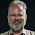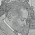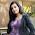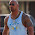Tuesday, March 11, 2014

Long Run Growth and Functional Finance

Tom Michl has some comments -- insightful as always -- on the previous post on functional finance. The key point he raises is that we can't treat the long-run growth rate as given exogenously. Policy that targets current output will also have effects on the long run trajectory that have to be taken into account. He writes:
I've become convinced that the real interest rate belongs in the investment equation, g(r), which means that g-r is not something we can just set greater than zero to solve the problem of fiscal deficits. Also that fiscal policy, e.g. the debt ratio, affects the long run position of the IS curve (assuming interest payments go to rentiers who spend them on consumption), so it affects the r that stabilizes inflation.
The question of exo/endogenous growth is important because a given growth target puts constraints on the feasible fiscal policy, or given fiscal policy, on the ability of a monetary authority to set the inflation-neutral interest rate.
This is something I've heard from other smart people in response to these arguments.  I certainly agree with Tom and everyone else that a complete story cannot just take g as given. And I agree that there should be some systematic relationship between the liquidity conditions that we summarize as the interest rate and demand conditions, and the long term growth of income. But it doesn't seem so straightforward to show that this relationship will be a constraint on the fiscal position.

There are two (sets of) channels: The one Tom mentions here, from financial conditions to investment to growth, and the other, on the supply side from the output gap via the labor supply (hysteresis) or technological change (Verdoorn's law) to growth. I think the second kind of channel is very important, but it doesn't create any issues for a functional finance position. It just means that we should define a higher level of output as "full employment" or "price stability." So let's focus on the first channel.

We think of investment as additions to the capital stock. Then we have g = s/c - dk, where g is the growth rate, s is the average savings rate, c is the incremental capital-output ratio, d is the depreciation rate and k is the average capital-output ratio. This is just accounting. As we know, this accounting relationship is often used to develop the idea of knife-edge instability. But that's never seemed right to me (and I don't think it's what Harrod intended with it.) s is the average savings rate, so in a Keynesian framework it will be a negative function of output. So what this equation is telling us is that if we need to achieve a given growth rate, and the capital-output ratio is fixed, then the output gap will have to adjust so as to get s to satisfy this equation. This is the adjustment that policy is allowing us to avoid. Fiscal policy raises or lowers average s at a given level of income. Monetary policy perhaps raises or lowers s also; more conventionally it is supposed to change the desired capital-output ratio c

So from my point of view, it is not quite correct to say that growth is a function of the interest rate. Rather, variation in the interest rate allows us to reconcile full employment with our chosen growth rate, whatever it may be.

Now, if we think that interest rates act through the capital-output ratio, then we need that as an additional degree of freedom if we want to combine full employment, our chosen growth rate, and a stable debt-income ratio. As it happens, Peter Skott and Soon Ryoo presented a paper at the Easterns where the requirement to achieve a target capital-output ratio meant that monetary policy was not available to close the output gap, requiring the use of fiscal policy. The additional degree of freedom is supplied by allowing the debt-GDP ratio to evolve freely.  This isn't a problem in this case. In their model, fiscal policy works through its additions to the stock of assets available to private wealth-owners, not through the flow of demand for currently produced goods and services. So the public-debt GDP ratio will automatically converge to whatever level satisfies the private sector's demand for net wealth above the capital stock.

So Peter's paper, I think, addresses Tom's point. Yes, fiscal policy affects the IS curve. Namely, it moves the IS curve to wherever it needs to be to get full employment at a given growth rate, as long as we are willing to let either the ratio of either capital or debt to output vary endogenously.

The bottom line is that when you move from the short run to the long run you do have to think about growth but that does not necessarily impose any additional constraint. First, if monetary policy operates through the savings rate, then the price stability target already implicitly means price stability at a given growth rate. So the model works the same regardless of what you think the growth rate is or should be. If monetary policy works through the capital-output ratio, then we can no longer say that, but in that case the capital-output ratio itself provides an additional degree of freedom. Only if we impose both a given growth rate and a given capital-output ratio do we possibly foreclose the option of setting r at whatever value is consistent with price stability and a constant debt ratio. And even then, I emphasize "possibly," because it depends on what you think happens to maintain the constraint. It seems to me the most natural answer is that at some point the desired capital-output ratio becomes insensitive to the interest rate, so, assuming that savings are also insensitive, then full employment cannot be reached at our target growth rate through monetary policy alone. In that case, fiscal policy becomes mandatory. This is where Keynes ended up, more or less, and also the implication of Peter's paper. But this doesn't give any argument for why interest rates cannot stay arbitrarily low, it just says that even very low interest rates won't be sufficiently expansionary to get us to full employment at low growth rates and that fiscal policy or some equivalent will be required as well. Which, as they say, is where we came in.

(I realize that this post probably will not make sense unless you're already having this conversation.)

 Last year's functional finance post is, thanks to my coauthor Arjun Jayadev, evolving into an academic article; I presented a version at the Eastern Economic Association a few days ago. This was one of the main comments there.

1.Hey JW: we want moar posts!

But I can't just write a comment so short, so I'll try to say something about functional finance and growth.

So, my point is: the real interest cannot be much lower than the real average profit rate, because, if it is much lower, a lot of people would borrow and invest in capital stuff and make a lot of money, until they reach the "end of possible productive investiment" point, lowering the average profit rate to the real interest rate. Let's say for ease that the real interest rate is i = k p, where k is a coefficient close to 1 and p is the real profit rate.

We know that i <= g is necessarious to have a sustainable debt path.

This means that in pratice to have a sustainable debt path (private or public) we need that:

p <= g/k
(since k is close to 1 this means p <= g).

That is, the real rate of profit has to be lower or equal to the real rate of growth or we will incur in a financial crisis.

Is this correct?

Also, intuitively I think that if p<=g the share of income that goes into wages has to be stable or growing, whereas if the share of income that goes into profits is growing it means that p>g, which sorta explains why we had a really big financial meltdown.

Is this correct?

1.A few responses.

In the real world we should expect profit rates on new investment to be higher than interest rates. High profit sectors always have some degree of monopoly power that will limit new investment, many productive enterprises are credit-constrained to some degree, and embodying capital in the form of fixed assets always involves costs and risks above those of holding it in money form. I've seen estimates of average industrial profit rates in 19th century Britain being on the order of 10 percent, implying a value for your k of less 0.5. However, the broader point is still legitimate. This was Wicksell's big idea -- that the "natural" rate of interest had some definite relationship to the expected profit rate on new investment.

If we adopt the assumption that all profits are reinvested and there is no investment out of wage income (the assumption of the classical economists, including Marx), then the profit rate and the growth rate are not two independent variables. Rather, the gross profit rate will be equal to the growth rate plus the depreciation rate plus the change in the capita-output ratio. Here we are talking about the realized aggregate profit rate, rather the expected marginal rate. In this story there is no role for the interest rate as an independent determinant of investment.

To have the profit rate net of depreciation persistently above the growth rate requires that either the profit share is increasing, or the capital-output ratio is decreasing. Both are logically possible, tho the latter seems unlikely. On the other hand, it's not clear that net of depreciation is the right way to think of this, since depreciation depends mainly on competition rather than any kind of physical wearing out of means of production. Then we can have the gross profit rate rise relative to the growth rate with no implications for distribution, if the depreciation rate also rises. This kind of more rapid scrapping of old equipment during a profit boom is a regular feature of the history of capitalism.

I'm sorry this isn't a very coherent answer. I am trying to figure this stuff out also.

2.I have two nitpicks:
1) I wrote "real average profit rate", and I forgot to write the footnote. But of course it is the rate of profit on the marginal capital that counts. However, where high profit sectors have monopolies, I think that those monopolies are generally reflected in the valutation of their "immaterial capital", so that while their material capital has an higer rate of profit, their total financial assets are higer so that their final rate of profit is the same.

2) You say that a decreasing capital-output ratio is unlikely. This is true in material terms, but in price terms it is not so unklikely.
For example, suppose that car factories have big and increasing scale efficiencies. Every car maker will try to undercut its competitors by lowering car prices and stal market shares. But then the price of cars fall vs. the cost of capital. In this situation every carmaker sujectively faces increasing returns on capital investiment, but the car making industry as a whole will face decreasing rates of profit.

2.we want moar posts!

3.If you not hanging out at Zabars already alongside the grey-ing eminence;
http://www.huffingtonpost.com/2014/02/28/paul-krugman-retire-princeton_n_4873500.html

1.Yes, it's very good news. (Tho I'm more of a Fairway person.)

4.Its a better idea to grow in the market with financial functional to be considered and make a great sign in the market turned.

Free Stock Market Tips on Mobile

5.